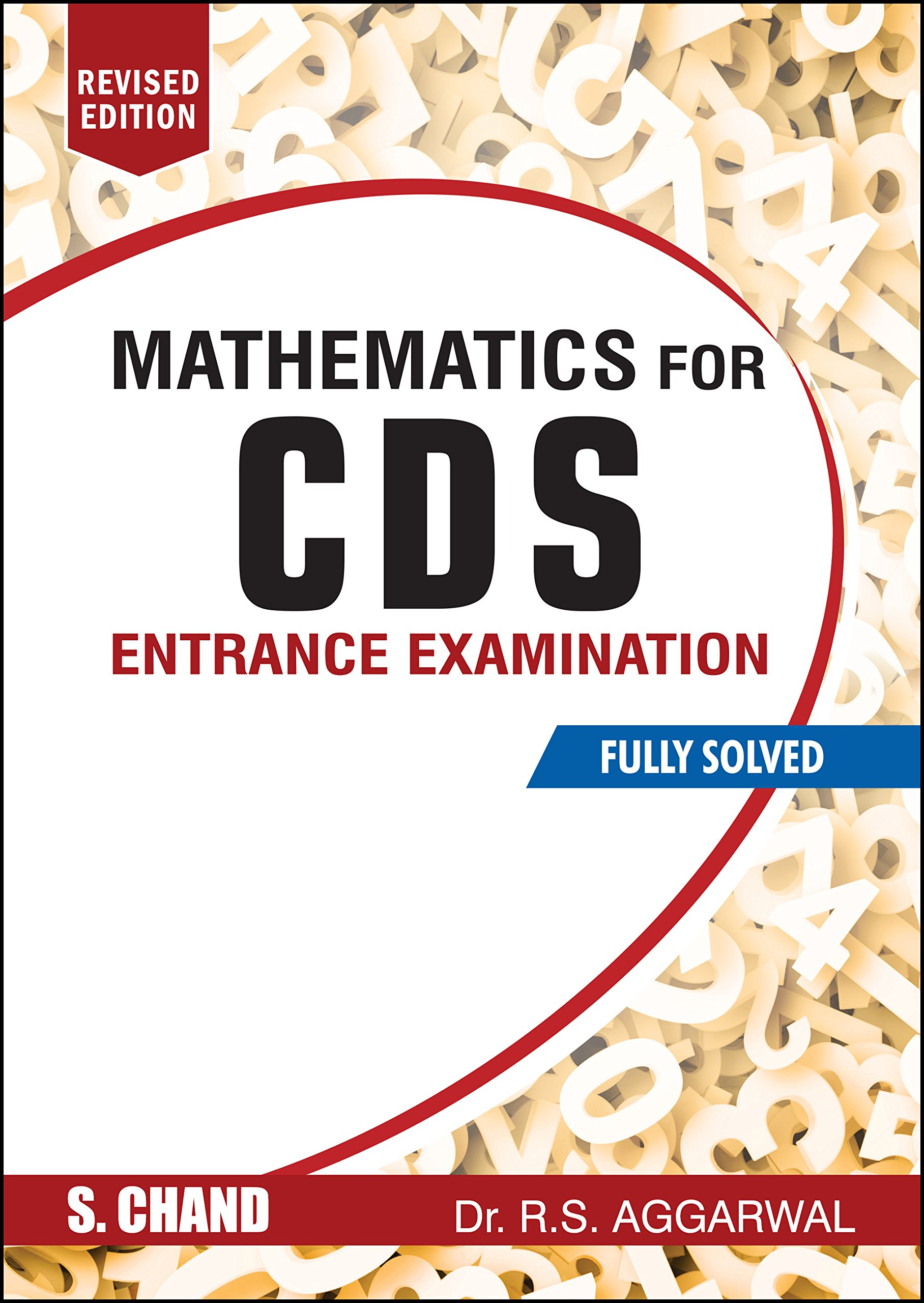# Mathematics for CDS Entrance Examination Fully Solved by R.S. Aggarwal (Revised Edition)Price: ₹ 350.00
(as of Sep 20,2021 10:59:37 UTC – Details)

Mathematics for CDS Entrance Examination retains its key strength in offering to students a huge accumulation of objective type questions with their solutions, while adding new questions to help students understand the latest pattern and trend of questions asked in recent examinations. These additional questions and their solutions, at the end of each chapter, from as recent as 2017 CDS exam would definitely help candidates prepare for the test better and infuse confidence among them.

Key Features:
• Fully solved examples with explained techniques.
• A huge collection of practicable questions, fully solved.
• References of questions asked in various Competitive Examinations, on memory basis.

Table of Content :

ARITHMETIC
• Numbers • H.C.F. and L.C.M. of Numbers • Decimal Fractions • Square Roots • Percentage • Average • Ratio and Proportion • Partnership • Profit and Loss • Unitary Method • Time and Work • Time and Distance • Problems on Ages • Simple Interest • Compound Interest • Logarithms

MENSURATION
• Area • Volume and Surface Areas

ALGEBRA
• Basic Operations and Factorization • H.C.F. and L.C.M. of Polynomials • Linear Equations • Quadratic Equations • Rational Expressions • Surds and Indices • Set Theory

TRIGONOMETRY
• Identities and T-Ratios • Height and Distance

GEOMETRY
• Lines and Angles • Polygons • Triangles • Quadrilaterals and Parallelograms • Circles • Loci

COORDINATE GEOMETRY
• Coordinate Geometry

STATISTICS
• Statistical Data (Frequency distribution, Mean, Mode, Median and Standard Deviation) • Data Interpretation